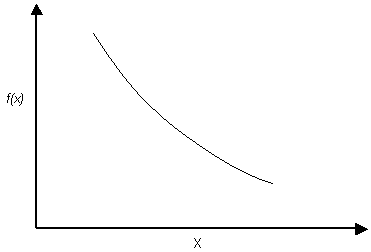Expert Modelling: Hands-on help from Rocscience Engineers. Register Here

# Exponential Distribution

The Exponential probability density function is available in SWedge.Exponential probability density function

NOTES:

• The range of values must always be positive for an Exponential distribution, therefore it should not be used for orientation distributions which include negative values (e.g. Dip or Dip Direction values less than zero).
• The mean is always equal to the standard deviation for an Exponential distribution. This is a property of the Exponential distribution, and cannot be altered by the user.
• Like the Normal distribution, the Exponential distribution can be truncated by entering the desired minimum and maximum values (the basic Exponential distribution varies from zero to infinity).

The Exponential distribution is sometimes used to define events, such as the occurrence of earthquakes or rockbursts, or quantities such as the length of joints in a rock mass. Of the currently defined statistical variables in SWedge, you may find an exponential distribution useful for modeling joint persistence, waviness angle, or cohesion, for example.